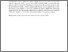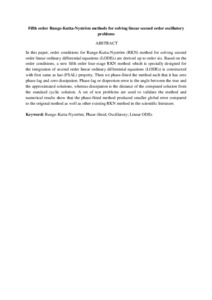# Fifth order Runge-Kutta-Nyström methods for solving linear second order oscillatory problems

## Citation

Salih, Mohammed M. and Ismail, Fudziah and Senu, Norazak (2016) Fifth order Runge-Kutta-Nyström methods for solving linear second order oscillatory problems. Far East Journal of Applied Mathematics, 95 (2). pp. 141-156. ISSN 0972-0960

## Abstract

In this paper, order conditions for Runge-Kutta-Nyström (RKN) method for solving second order linear ordinary differential equations (LODEs) are derived up to order six. Based on the order conditions, a new fifth order four-stage RKN method which is specially designed for the integration of second order linear ordinary differential equations (LODEs) is constructed with first same as last (FSAL) property. Then we phase-fitted the method such that it has zero phase-lag and zero dissipation. Phase-lag or dispersion error is the angle between the true and the approximated solutions, whereas dissipation is the distance of the computed solution from the standard cyclic solution. A set of test problems are used to validate the method and numerical results show that the phase-fitted method produced smaller global error compared to the original method as well as other existing RKN method in the scientific literature.Preview
Text
Fifth order Runge-Kutta-Nyström methods .pdfView Item